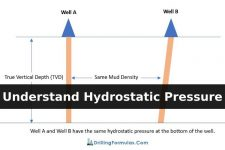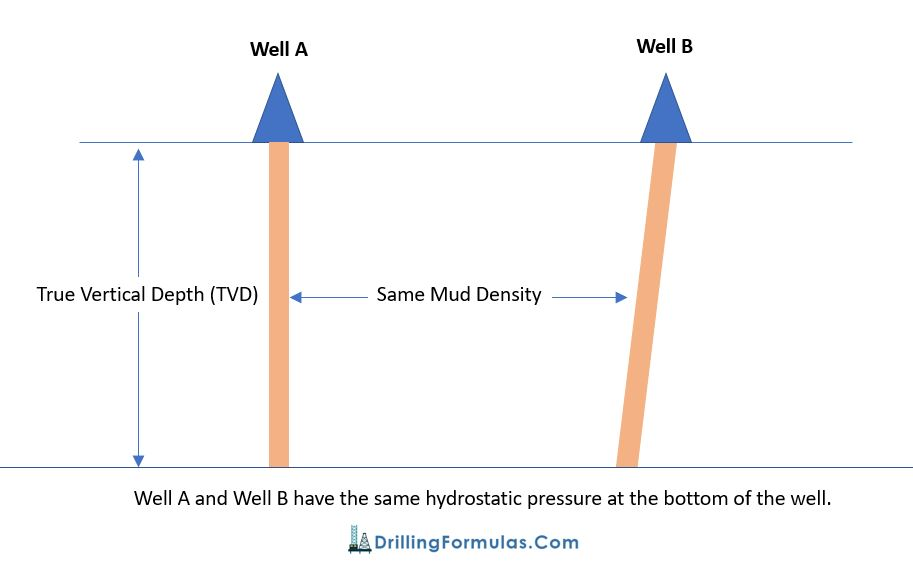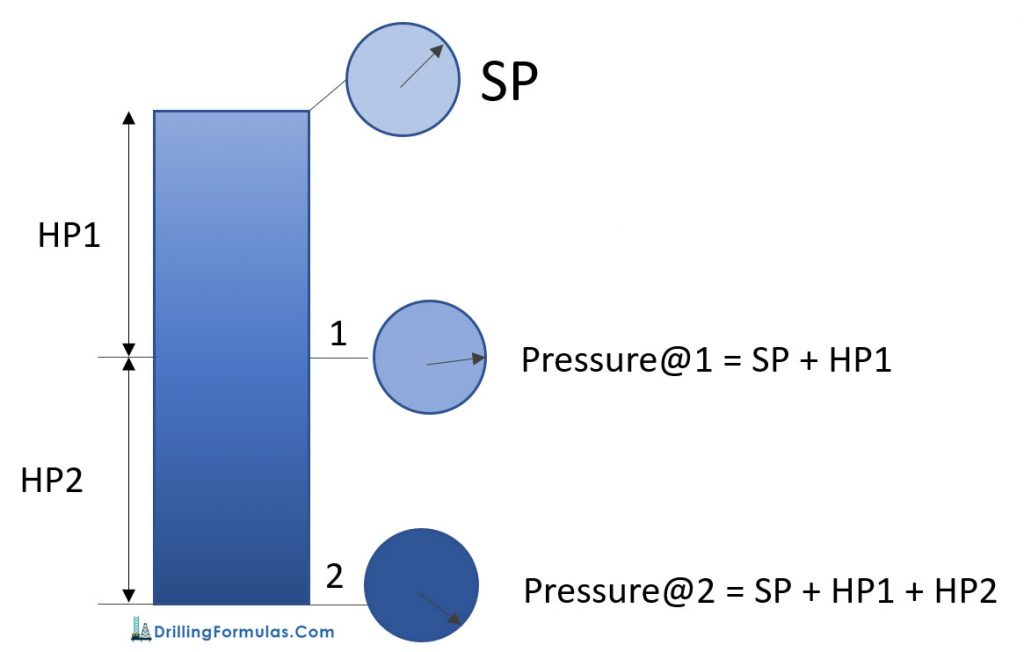## Understand Hydrostatic PressureIn a well, any pressure created by a static column of fluid is called ‘Hydrostatic Pressure’ (HP); at any given True Vertical Depth (TVD). With ‘hydro’ representing water, which exerts pressure, ‘static’ means it has no movement. Any pressure developed by a column of fluid that isn’t moving, therefore, can be considered hydrostatic pressure; fluid in this sense can be either liquid or gas.

The relationship of hydrostatic pressure is shown in the equation below.

HP (Hydrostatic Pressure) = density x g (gravity acceleration) x h (True Vertical Depth, TVD)

In oilfield term, the formula above is modified so that people can use it easily. The formulas are as follows:

HP (Hydrostatic Pressure) = Constant x MW (Mud Weight or Mud Density)  x TVD (True Vertical Depth)

HP (psi)  = 0.052 x MW (ppg) x TVD (ft) ** Most frequent used in the oilfield **

HP (psi) = 0.007 x MW (pcf) x TVD (ft)

HP (kPa) = 0.00981 x MW (kg/m3) x TVD (m)

Depending on which unit is used for calculation, there are several conversion factors such as 0.052, 0.007, 0.00981 for instant as you can see from the equations above.

According to the equations above, Hydrostatic Pressure is not a function of hole geometry. Only mud weight and True Vertical Depth (TVD) affect on Hydrostatic Pressure. For example (a picture below); well A and well B have the same vertical depth. With the same mud density in hole, the bottom hole pressure due to hydrostatic pressure is the same. The only different between Well A and Well B is mud volume.This concept is basic and very important for many aspects such as well control, balance cementing, u-tube, etc.

You can learn more about hydrostatic pressure calculation from the following article – Hydrostatic Pressure Calculation

## Pressure in a well

In a static condition

• Pressure at any depth = Hydrostatic Pressure (HP) + Surface Pressure (SP)
• Pressure between 2 points is HP between these points

The diagram below demonstrates the relationship of pressure in a well.

At point 1, Pressure@1 = Surface Pressure (SP) + Hydrostatic Pressure @ 1 (HP1)

At point 1, Pressure@2 = Surface Pressure (SP) + Hydrostatic Pressure@1 (HP1) + Hydrostatic Pressure@2 (HP2)Ref books:

Lapeyrouse, N.J., 2002. Formulas and calculations for drilling, production and workover, Boston: Gulf Professional publishing.

Bourgoyne, A.J.T., Chenevert , M.E. & Millheim, K.K., 1986. SPE Textbook Series, Volume 2: Applied Drilling Engineering, Society of Petroleum Engineers.

Mitchell, R.F., Miska, S. & Aadny, B.S., 2011. Fundamentals of drilling engineering, Richardson, TX: Society of Petroleum Engineers.